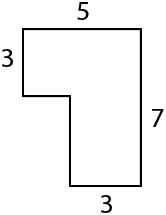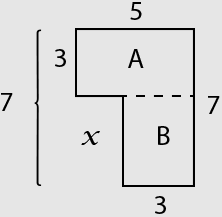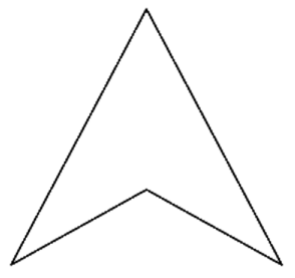# TABE Math Practice Test: Level E

Below is our TABE 11 & 12 Math practice test for Level E. This is the easiest of the math levels, so this is a good place to start. If you find these questions to be too easy, go ahead and move on to Math Level M. Topics on this test include basic arithmetic, measurement, fractions, geometry, and algebraic thinking. Start your test prep now with this free TABE Math practice test!

Congratulations - you have completed .

You scored %%SCORE%% out of %%TOTAL%%.

Your performance has been rated as %%RATING%%

 Question 1

### Look at the figure below.### What is the area of this shape?

 A 35 B 36 C 30 D 27
Question 1 Explanation:
The correct answer is (D). Begin by splitting the shape into two rectangles:The area of a rectangle is the product of its length and width:

$A_\text{Rect} = l \ast w$

For rectangle A we have:

$A_\text{RectA} = 5 \ast 3 = 15$

For rectangle B we need to find the missing dimension, represented by $x$. We know that 3 + $x$ = 7 . Thus, $x$ = 4. The area of rectangle B is:

$A_\text{RectB} = 3 \ast 4 = 12$

The total area of our shape is the sum of the areas of rectangles A and B:

$A_\text{total} = 15 + 12 = 27$
 Question 2

### Randy is collecting donations for a fund-raiser. So far he has received four donations: \$48, \$15, \$33, \$25. How much money has been donated in total?

 A \$120 B \$121 C \$117 D \$111
Question 2 Explanation:
The correct answer is (B). Add the four amounts to calculate the total:Question 3

### Which of the following fractions is greatest? $\dfrac{4}{5},\dfrac{3}{7},\dfrac{11}{24},\dfrac{2}{3}$

 A $\dfrac{2}{3}$ B $\dfrac{3}{7}$ C $\dfrac{4}{5}$ D $\dfrac{11}{24}$
Question 3 Explanation:
The correct answer is (C). As a general strategy, begin by determining if each fraction is greater that, less than, or equal to one-half.

Compare the numerator to one-half of the denominator:

$\dfrac{4}{5}$: 4 is greater that 2.5 (half of 5), so $\frac{4}{5}$ is greater than $\frac{1}{2}$.

$\dfrac{3}{7}$: 3 is less than 3.5 (half of 7),so $\frac{3}{7}$ is less that $\frac{1}{2}$.

$\dfrac{11}{24}$: 11 is less than 12 (half of 24), so $\frac{11}{24}$ is less than $\frac{1}{2}$.

$\dfrac{2}{3}$: 2 is greater that 1.5 (half of 3), so $\frac{2}{3}$ is greater than $\frac{1}{2}$.

Only two of the fractions are greater than $\frac{1}{2}$. Either $\frac{4}{5}$ or $\frac{2}{3}$ is the greatest fraction.

To determine which is greatest, it is probably easiest to think about the "size of the missing piece".

If you have a pizza cut into 5 slices and another pizza cut into 3 slices, each of the 5-slice pieces is going to be smaller than each of the 3-slice pieces.

So, if you have 4 slices out of the 5-slice pizza, the one piece you did not get (the "missing piece") is smaller than the one piece missing from the 3-slice pizza.$\; \dfrac{4}{5}$ is greater than $\dfrac{2}{3}$
 Question 4

### Lea has \$250. She spends \$172 on gifts for friends. How much money does she have left?

 A \$88 B \$188 C \$78 D \$82
Question 4 Explanation:
The correct answer is (C). Subtract 172 from 250:Question 5

### Jill is taking inventory in her warehouse. For Item A she counts 60 boxes containing 8 pieces per box. For Item B she counts 90 boxes containing 5 pieces per box. Which of the following correctly compares the amounts she counted.

 A 480 > 450 B 480 > 420 C 480 < 420 D 480 < 450
Question 5 Explanation:
The correct answer is (A). Calculate the totals for each item.

Item A:

$8 \ast 60 = 8 \ast 6 \ast 10$ $= 48 \ast 10 = 480$

Item B:

$5 \ast 90 = 5 \ast 9 \ast 10$ $= 45 \ast 10 = 450$

She counted 480 and 450.

Only answer choices (A) and (D) contain both of the numbers. Only (A) shows a valid statement, 480 is greater that 450. Remember: "the mouth eats the larger number."
 Question 6

### Al, Bob, Charlie, and Doug combined the contents of their meager savings to raise enough money to see a movie together. Al had \$5, Bob had \$8, and Charlie had \$9. Each movie ticket cost \$7. How much did Doug contribute?

 A \$8 B \$7 C \$5 D \$6
Question 6 Explanation:
The correct answer is (D). To see the movie the movie together, they need four tickets. Each ticket costs \$7.$4 \ast \$7 = \$ 28$They needed \$28 to see the movie together. We know the amounts contributed by three of the friends, but the fourth amount is unknown. We can set up the following equation to represent the situation:

$\$5 + \$8 + \$9 + x = \$28$

Simplifying:

$\$22 + x = \$28$

Solving:

$( 22 \; + \; ? = 28):$

$x = \$6$ Question 7 ### Tricia’s company receives the following shipment from its supplier.### She needs to repackage them into boxes of 8 pieces per box. How many boxes will she need?  A 6 B 5 C 4 D 7 Question 7 Explanation: The correct answer is (B). Each bundle contains 10 rods. There are 4 bundles. This means we have:$4 \ast 10 = 40 \text{ rods}$If they are repackaged into boxes of 8, we will need:$\dfrac{40}{8}=5 \text{ boxes}$ Question 8### The shape shown above can be correctly described by which of the following?  A Trapezoid B Rhombus C Quadrilateral D Parallelogram Question 8 Explanation: The correct answer is (C). • The given shape has four sides making it a quadrilateral (quad = four and lateral = side). None of its sides are parallel, so the only possible answer is (C). • A Trapezoid is a quadrilateral with one set of parallel sides. • A Rhombus is a quadrilateral with two sets of parallel sides, making it a parallelogram, and four sides of equal length. • A Parallelogram is a quadrilateral with two sets of parallel sides.Question 9 ### Micah started from home and arrived at the cabin at 1:23 PM. The drive to the cabin took 150 minutes, and he made no stops along the way. If his home and the cabin are in the same time zone, what time did he leave his home?  A 10:53 AM B 11:23 AM C 3:53 PM D 11:33 AM Question 9 Explanation: The correct answer is (A). 150 minutes = 60 + 60 + 30 minutes = 2 hours and 30 minutes. He left his home 2 hours and 30 minutes before 11:23 PM:He left his home at 10:53 AM.  Question 10 ### The large cylindrical container shown in the figure below has markings indicating the number of liters of liquid it contains. If the liquid contents of the container are split equally between two barrels, how much liquid will each of the barrels contain? ###A 32 B 34 C 16 D 17 Question 10 Explanation: The correct answer is (D). We must first determine how much liquid is in the cylindrical container shown. Because there are only 5 graduations (marks) between 30 and 40, each graduation represent 2 liters. Our cylinder contains 34 liters. The 34 liters is split equally between two barrels:$\dfrac{34}{2}=17 \text{ liters}\$

Note: You can think of "half of 34" as "half of 30 plus half of 4" or 15 + 2 = 17. Each barrel will contain 17 liters.
Once you are finished, click the button below. Any items you have not completed will be marked incorrect.
There are 10 questions to complete.
 ← List →